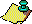Contents Next: The Floating-Point Gauss Up: Classical Results Interpreted Previous: Remark.

The Gauss map is well-known in ergodic theory (see  or ). The results are summarized here, for contrast with the results of the sections previous and following. This section is meant more as incentive for the reader to investigate ergodic theory than as exposition.

The Gauss map preserves the Gauss measure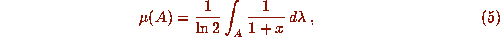where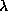is the Lebesgue measure. Thus the Gauss map is ergodic, and almost all (in the sense of either the Lebesgue or Gauss measure) initial points have orbits which have the interval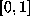as-limit set. Thus the only attractor whose basin of attraction has nonzero measure is the interval. By the ergodicity of the map, we may explicitly calculate the Lyapunov exponent as follows: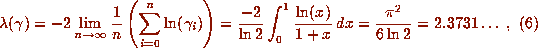which holds for almost all initial points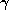. This is of interest, since there are few nontrivial maps for which the Lyapunov exponent can be calculated explicitly.

This section, more than any other in the paper, provoked puzzlement on the part of readers of the original. The original purpose was to show the reader how powerful ergodic methods were: in one line we establish the `almost-everywhere' value of the Lyapunov exponent for the Gauss map, using the ergodic result that the `time-average' of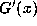along the orbit is equal to the (properly-weighted) `space-average' given by the integral of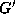with respect to the Gauss measure.

I have since also learned that this Lyapunov exponent can be explicitly connected with Khintchin's constant (mentioned previously as the geometric mean of the partial quotients, which turns out to be the same for almost all x in). Khintchin's constant is K, where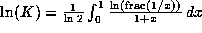. (By the same arguments). The only difference is the presence of the `fractional part'. This integral gives a simple convergent series for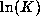which can be transformed into a quickly convergent one. (The integral becomes a series because frac(1/x) is piecewise constant). See  for details.Contents Next: The Floating-Point Gauss Up: Classical Results Interpreted Previous: Remark.